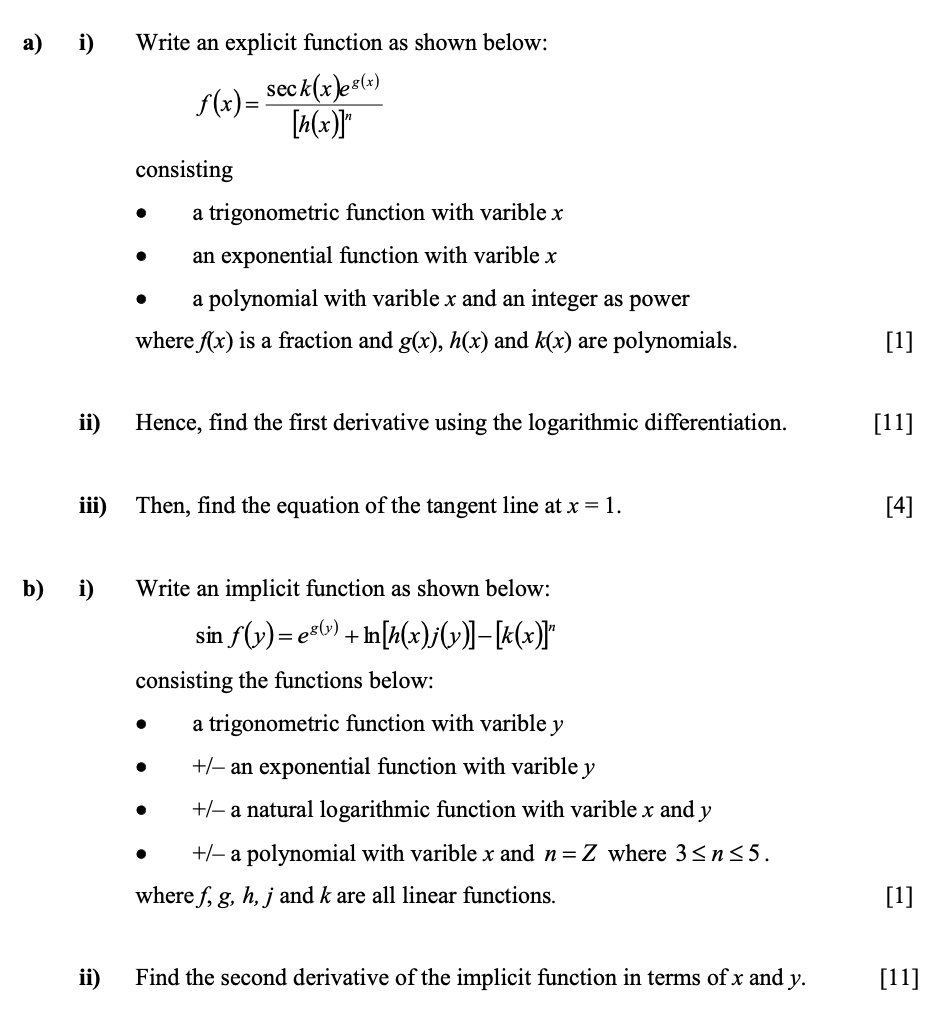💬 👋 We’re always here. Join our Discord to connect with other students 24/7, any time, night or day.Join Here!

IS

Isaac S.

Calculus 1 / AB

1 week, 5 days ago

# Write an explicit function as shown belowYale University

\begin{equation} \begin{array}{l}{\text { F. NOCHANGE }} \\ {\text { G. tells us, that, }} \\ {\text { H. tells us that, }} \\ {\text { J. tells us that }}\end{array} \end{equation}

### Discussion

You must be signed in to discuss.

### Video Transcript

So this grammatical question is about punctuation, and we want to make sure that punctuation follows the correct grammar rules. Eso if we look at the underlying passage, we actually don't need a comma because we wouldn't normally pause at that point of the passage. So since it is a necessary, we're going to go with an answer choice that eliminates tach, um, calm or any punctuation, which is J so answer. Choice Jay is correct, and the reason is no punctuation.Yale University
##### Top Calculus 1 / AB Educators##### Catherine R.

Missouri State University##### Kristen K.

University of Michigan - Ann Arbor##### Michael J.

Idaho State University